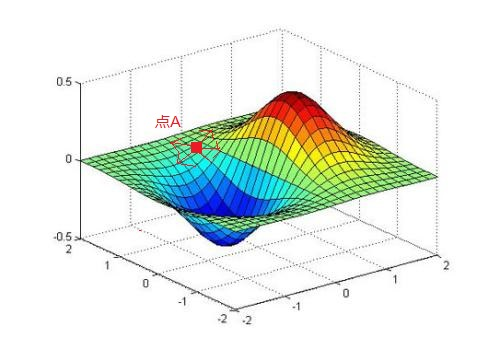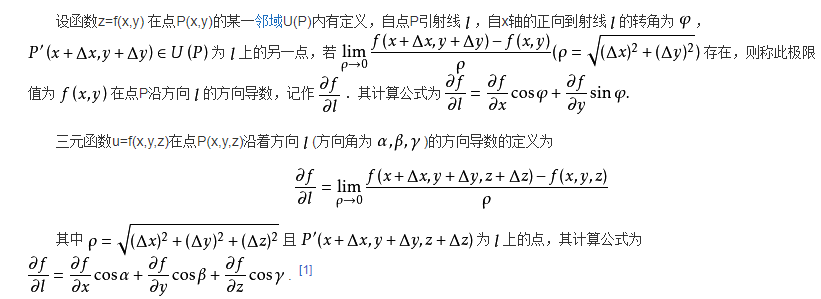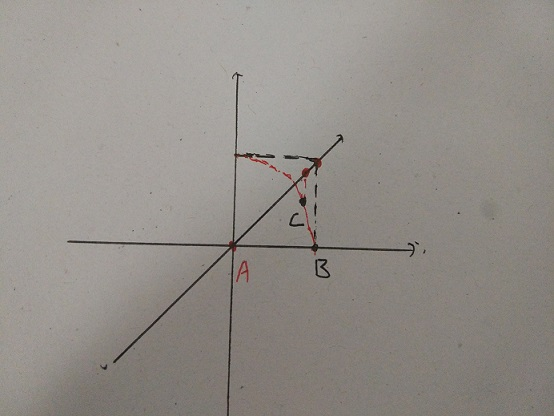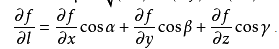GDBT--理解梯度提升原理篇

${f}_{M}\left(x\right)=\sum _{m=1}^{M}T\left(x;{Q}_{m}\right)$

什么是方向导数${F}^{\prime }=\frac{F\left(x+△x,y+△y,z+△z\right)-F\left(x,y,z\right)}{|\left(△x,△y,△z\right)|}$为什么负梯度方向是函数下降最快方向$\frac{⅁f}{⅁l}=\left(\frac{⅁f}{⅁x},\frac{⅁f}{⅁y},\frac{⅁f}{⅁z}\right)\ast \left(cosa,cos\beta ,cosy\right)$$\frac{\Game f}{\Game l}=(\frac{\Game f}{\Game x},\frac{\Game f}{\Game y},\frac{\Game f}{\Game z}) * (cosa,cos \beta,cos y)$
$|\frac{⅁f}{⅁l}|=|\left(\frac{⅁f}{⅁x},\frac{⅁f}{⅁y},\frac{⅁f}{⅁z}\right)|\ast |\left(cosa,cos\beta ,cosy\right)|\ast cos\theta$$|\frac{\Game f}{\Game l}|=|(\frac{\Game f}{\Game x},\frac{\Game f}{\Game y},\frac{\Game f}{\Game z})| * |(cosa,cos \beta,cos y)|*cos \theta$

$L\left(y,{y}^{hat}={f}_{m-1}\left(x,y,z\right)+learnrate\ast \left(-\frac{⅁L}{{f}_{m-1}}\right)\right)$

${f}_{m}\left(x,y,z\right)={f}_{m-1}\left(x,y,z\right)+learnrate\ast \left(-\frac{⅁L}{{f}_{m-1}}\right)$